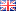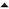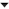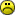## Facebook Hacker Cup 2016: Qualification Round problem analysisAs I'm writing this, the Qualification Round of the Facebook Hacker Cup 2016 is about to finish. For those not in the know: the Hacker Cup is an annual algorithmic programming contest, where contestants solve mathematical puzzles by writing some code (no "hacking" in the contemporary sense involved).

Over the past few years I've been posting my analysis of the problems on my blog, and I thought I might as well continue the tradition. As usual, there are major spoilers ahead, as well as code, and some bonus questions for those that managed to solve all the problems already. So let's begin!

#### Problem A: Boomerang Constellations

Problem statement (official link)

A boomerang constellation is a triple p-q-r such that the distance between p and q equals the distance between q and r. We're given the locations of the stars and are asked to count all the boomerang constellations.

Note that we don't have to enumerate all possible constellations; we just have to count them. Here's an idea on how to do that. Let's call the common endpoint (q in the triple p-q-r) the center of the constellation. For each potential center star, we can group the surrounding stars by equal distance to q, and then the only way to create a boomerang constellation is to pick the other two endpoints from a single group of equidistant stars.

For example, consider the beautiful artist's rendition of the night sky below:

```        d
|
a  |  b
\ | /
g - - - q - - - e
|
c
|
f
```

Here, point q is surrounded by two groups of stars: {a, b, c} (at distance 1) and {d, e, f, g} (at distance 3). To build a boomerang constellation with q at the center, we must pick the other two stars either from the first group (there are three possible pairs: {a, b}, {b, c} or {a, c}) or from the second group (with six possible pairs: {d, e}, {d, f}, {d, g}, {e, f}, {e, g}, {f, g}). That means there are 3 + 6 = 9 boomerang constellations with q in the center.

In general, there are n*(n - 1)/2 ways to select a pair of points from a set of n elements: we have n options to select the first point, then n - 1 options for the other point (one less, because we cannot pick the same star twice). Finally, we divide by 2 because pairs are unordered: a-q-b and b-q-a are really the same constellation.

Now all we need to do is consider all possible center points in turn, calculate the distances to the surrounding points, group them by distance, and add up all the combinations. This solution will take O(N2) time and O(N) space.

If we try to code it up in Python, the solution looks something like this:
Python:
 ```1 2 3 4 5 6 7 8 9 10 11 12 13 14 15 16 17 18 19 20 21 ``` ```from collections import defaultdict import sys def Solve(points): total_count = 0 for (x1, y1) in points: groups = defaultdict(int) for (x2, y2) in points: groups[(x1 - x2)**2 + (y1 - y2)**2] += 1 for count in groups.values(): total_count += count * (count - 1) / 2 return total_count T = int(sys.stdin.readline()) for t in range(T): N = int(sys.stdin.readline()) points = [] for _ in range(N): x, y = map(int, sys.stdin.readline().split()) points.append((x, y)) print "Case #{}: {}".format(t + 1, Solve(points))```

Note that the code above uses the square of the distance between points (instead of the actual distance). It's easier that way: squares of distances are always integers, so we don't have to worry about floating point inaccuracies, and grouping stars by distance squared works just as well.

Alternative approach: if you're using a reasonably efficient programming language like C++ or Java, then it's also possible to search over all triples (p,q,r) directly. Simply check for each triple whether the distances p-q and q-r are equal, and increment the answer if they are. This brute-force solution takes O(N3) time instead, but with N <= 2000, it just might work, and is even easier to code up.

#### Problem B: High Security

Problem statement (official link)

For the second problem, we're asked to place guards on a rectangular grid so that they can watch all open spaces. This easiest way to solve this problem is with a greedy algorithm, which is an algorithm that builds a solution incrementally based on local heuristics, without reconsidering decisions later (as in a backtracking algorithm).

The hard part about coming up with a greedy solution, is to prove that one exists at all! Fortunately, we can derive one, but we need to make two observations.

Let's consider maximal stretches of horizontal open spaces. First, let's consider stretches of width 1. These are single spaces with a wall directly to the left and right. To cover those, we must place a guard either on the space itself, or in the same column on the opposite row. The first essential observation for this problem, is this: if it's possible to place the guard on the opposite row of a single-width stretch, we must always do it!

For example:

```XX.XX  ->  XX.XX
.....      ..G..

X.XXX  ->  XGXXX
XXX.X      XXXGX

XX.XX  ->  XX.XX
XX.XX      XXGXX
```

In the first case, the guard on the second row can see both the 1-width stretch on the top row, and the rest of the bottom row. In the second case, the single-width stretches are surrounded by walls completely, so we have no choice to put a guard inside each of them. In the third case there are two single-width stretches facing each other; in that case, we can place a guard in either one of them.

If we greedily place guards this way, we'll eventually cover all the single-width stretches. That means the only uncovered squares remaining are part of a stretch of size greater than 1. The second essential observation is that for those remaining stretches, there is no better solution than to place a guard in each of them. We can see that this is true by observing that every multiple-cell stretch must either have a wall blocking one of the cells on the opposite row, or it must share at least two open spaces with a stretch on the other side:

```X...X      X.G.X
..X..  ->  G.X.G

....   ->  G...
....       G...

X..X       XG.X
X..X   ->  XG.X
```

In the first case above, each of the three stretches has at least one open space blocked by a wall, and that space can only be seen by a guard in the same stretch. In the second case, the stretches have four overlapping spaces. Clearly the best solution is to place one guard on each row. The third case is a bit of an edge case: there, we could put the two guards on the same row if we wanted to. However, the assumption still holds: putting one guard on each row is just as good.

Since we must place exactly one guard per remaining stretch, it clearly doesn't matter where we put them within the stretch, because each guard will always cover all squares on the same row. We might as well drop them on the first square of each stretch.

Now that we know how we can place the guards in an optimal configuration, writing the code to do so is easy. As an implementation detail, it's useful to place an extra wall at the beginning and end of each row. This allows us to scan through the rows looking for walls without checking if we've reached the edge of the grid:

Python:
 ```1 2 3 4 5 6 7 8 9 10 11 12 13 14 15 16 17 18 19 20 21 22 23 24 25 26 27 28 29 30 31 32 33 34 35 36 37 38 39 40 41 42 43 44 45 ``` ```import sys def Solve(N, lines): # Each element of grid will be one of: # '.' an open square (not seen) # '*' a square (seen by a guard) # 'G' a guard # 'X' a wall grid = [ ['X'] + list(line) + ['X'] for line in lines ] def MarkSeen(row, col): if grid[row][col] in 'GX': return False grid[row][col] = '*' return True def PlaceGuard(row, col): if grid[row][col] == 'X': return False grid[row][col] = 'G' MarkSeen(1 - row, col) left = col - 1 while MarkSeen(row, left): left -= 1 right = col + 1 while MarkSeen(row, right): right += 1 return True # Pass 1: place guards to cover all single-width stretches. for row in range(2): for col in range(1, N + 1): if grid[row][col - 1:col + 2] == ['X', '.', 'X']: PlaceGuard(1 - row, col) or PlaceGuard(row, col) # Pass 2: place one guard in each remaining stretch. for row in range(2): for col in range(1, N + 1): if grid[row][col] == '.': PlaceGuard(row, col) return sum(row.count('G') for row in grid) T = int(sys.stdin.readline()) for t in range(T): N = int(sys.stdin.readline()) lines = [ sys.stdin.readline().strip() for _ in range(2) ] assert map(len, lines) == [N, N] print "Case #{}: {}".format(t + 1, Solve(N, lines))```

The nice thing about a greedy solution is that it's constructive: the resulting grid contains the optimal arrangement of guards, instead of just calculating the minimal number. The code above runs in O(N) time. It's easy to accidentally create an O(N2) solution, but with N <= 1000, that would probably work, too.

Alternative approach: the problem can also be solved with dynamic programming. In that case, keep track of the next column to fill in, and whether there are any guards or open squares to the left (for each of the two rows). This also yields an O(N) solution, but the implementation is much trickier. I wouldn't recommended it, though it's a good alternative if you cannot come up with the greedy solution. If you're curious what that looks like, check out my solution in C++ here.

#### Problem C: The Price is Correct

Problem statement (official link)

We need to count all substrings of an array of positive integers, B, that have a sum less than or equal to some given number, P. This sounds simple enough, but here's the tricky part: B can contain up to 100,000 elements. This means we can't just consider all possible substrings, because there may be close to 5 billion of them, and that's just too much to brute-force. However, this is the kind of problem where it makes sense to start with a brute-force solution anyway, and then consider how we can improve it. It turns out that we can turn a slow solution into a fast one with just a few tweaks.

First, let's define S(i, j) as the sum of B[k] for all i <= k <= j. That means we need to count all pairs (i,j) with i <= j such that S(i, j) <= P.

Now, let's call a range [i:j] maximal if S(i, j) <= P and S(i-1, j) > P (or i = 1) and S(i, j+1) > P (or j = N). Intuitively, a range is maximal if we can't extend it to the left or right. For example, if P = 5, and B=[2,2,2,2], then [2:3] is a maximal range, because S(2,3) = 4 and the range cannot be extended to the left (S(1,3) = 6 > P) or right (S(2,4) = 6 > P).

Since all prices are greater than zero, that means that for a fixed i there is a unique j such that [i:j] is maximal, as the sum S(i, j) increases with j. Similarly, for each j there is a unique i. Also, if S(i,j) <= P then S(k,j) <= P for all i <= k <= j. That means that if we can find all maximal ranges, then we can find the total number of solution pairs by summing the lengths of the maximal ranges.

Now how do we find those maximal ranges? Let's assume that one endpoint, (say, j) is fixed. We can start with i=1, and keep incrementing i until S(i, j) <= P. Then, we've found our maximal range, and can add its size (j - i + 1) to the answer. In Python, this solution would look like this:

Python:
 ```1 2 3 4 5 6 7 8 9 ``` ```def Solve(N, P, B): answer = 0 for j in range(N): i = 0 while i <= j and sum(B[i:j + 1]) > P: i += 1 # Now, [i:j] is the maximal range ending at j answer += j - i + 1 return answer```

(Note that in Python, array indices start at 0 and the ending index of a range is exclusive, as GodDijkstra intended.) The code above produces the correct answer, but it's too slow: we execute the outer loop N times, and the inner loop N/2 times on average (in the worst case). As mentioned before, N is too large to allow an O(N2) solution to pass.

One way to speed it up is to observe that if S(i, j) is maximal, than means S(i-1, j) > P (or i=1). In that case, S(i-1, j+1) > P too, so instead of starting from 1 (or 0 in Python), we can reuse the previous value of i in the outer loop:

Python:
 ```1 2 3 4 5 6 7 8 ``` ```def Solve(N, P, B): answer = i = 0 for j in range(N): while i <= j and sum(B[i:j + 1]) > P: i += 1 # Now, [i:j] is the maximal range ending at j answer += j - i + 1 return answer```

Can you even spot the difference? This tiny change has a big impact: instead of N2/2 times, the inner loop is now executed at most N times, because i strictly increases from 0 to N. Unfortunately, the solution is still too slow, and the culprit is the expression sum(B[i:j + 1]) in the condition of the inner loop: calculating the sum of a range takes time proportional to the length of the range. To fix that, let's keep a running total of the sum and update it whenever we increment i or j, yielding the final version of the code:
Python:
 ```1 2 3 4 5 6 7 8 9 10 11 12 13 14 15 16 17 18 ``` ```import sys def Solve(N, P, B): answer = total = i = 0 for j in range(N): total += B[j] while i <= j and total > P: total -= B[i] i += 1 answer += j - i + 1 return answer T = int(sys.stdin.readline()) for t in range(T): N, P = map(int, sys.stdin.readline().split()) B = map(int, sys.stdin.readline().split()) assert len(B) == N print "Case #{}: {}".format(t + 1, Solve(N, P, B))```

The final solution is simple and fast, and runs in linear time and space.

#### Problem D: Text Editor

Problem statement (official link)

The final problem is a classical dynamic programming problem. Before we can start coding, we need to define the functions we want to calculate. Then, the actual implementation simply consists of tabulating the values of those functions.

Suppose we've already selected a set of K words. In what order should we output them to minimize the number of keystrokes? It turns out that alphabetical order is one of the optimal ways to do it (the proof is left as an exercise to the reader). With this observation, we can start by sorting the dictionary, and then consider subsequences of the dictionary in this fixed order only.

Now, let |w| denote the length of word w. Then, to output a single word w, we need |w| * 2 + 1 keystrokes: first |w| to type the word, then 1 to hit the print button, and finally another |w| backspaces to clear the editor buffer. Let's define a function InitialCost as follows:

`InitialCost(w) = 2*|w| + 1`

Okay, so supposed we have a sequence that ends with some word v, and we want to type a different word, w, right after v. Typing w would also take |w| * 2 + 1 keystrokes, but if v and w share a common prefix of length (say) l, then we can save 2*l keystrokes because we don't have to clear the entire buffer in between. In fact, we can save l backspaces and l letters of w. Let's define a function CommonPrefix that calculates the length of the longest common prefix of two strings. For example, CommonPrefix("foobar", "fooquux") = 3, because both words start with the 3-letter prefix "foo". With that, we can define the cost of outputting w after v as follows:

`ConcatCost(w, v) = InitialCost(w) - 2*CommonPrefix(v, w)`

Note that ConcatCost(w, v) equals the cost of concatening w to any sequence that starts with v (regardless of how long the existing sequence was) because all that matters is the last word in the buffer before we start typing the next.

Now, let Cost(k, w) denote the minimum number of keystrokes necessary to type a sequence of k words ending with word w. How can we implement that function? For k = 1, Cost(k, w) simply equals InitialCost(w). For k > 1, we can concatenate w to any sequence of length k-1 that ends with some word v that precedes it. More formally:

```Cost(1, w) = InitialCost(w)
Cost(k, w) = minimum ConcatCost(v, w) + Cost(k-1, v) for any v < w```

Here we're using the fact that we've restricted ourselves to alphabetically increasing subsequences of words. Without this restriction (i.e. without the v < w part in the definition of Cost above) we might reuse words in our output, which is not allowed.

Now that we've defined Cost, the answer we're ultimately looking for is the minimum Cost(k, w) for k=K over all w.

To implement this, we will start by sorting the dictionary. Then, we'll calculate the function ConcatCost and store the results for all arguments in an array. Finally, we'll calculate Cost. In Python:

Python:
 ```1 2 3 4 5 6 7 8 9 10 11 12 13 14 15 16 17 18 19 20 21 22 23 24 25 26 27 28 29 ``` ```import sys def CommonPrefix(v, w): n = 0 while n < len(v) and n < len(w) and v[n] == w[n]: n += 1 return n InitialCost = lambda v: 2*len(v) + 1 ConcatCost = lambda w, v: InitialCost(w) - 2*CommonPrefix(v, w) def Solve(N, K, words): words.sort() concat = [[ConcatCost(words[i], words[j]) for j in range(N)] for i in range(N)] cost = [[float('inf') for i in range(N)] for j in range(K)] for i in range(N): cost[i] = InitialCost(words[i]) for k in range(1, K): for i in range(1, N): cost[k][i] = min(concat[i][j] + cost[k - 1][j] for j in range(i)) return min(cost[K - 1]) T = int(sys.stdin.readline()) for w in range(T): N, K = map(int, sys.stdin.readline().split()) words = [ sys.stdin.readline().strip() for _ in range(N) ] print "Case #{}: {}".format(w + 1, Solve(N, K, words))```

So what's the complexity of this approach? Calculating the cost array takes O(N*K) time. The preceding two operations are a little harder to analyze. Sorting the array takes O(L*N log N) time, where L is the average common prefix length between pairs of strings. Similarly, calculating CommonPrefix(v, w) takes O(L) time on average, so calculating concat takes O(N2*L) time in total. Although it's possible to optimize the calculation of these functions further, the input for this problem (limited to 100,000 characters per testcase) is small enough that the obvious approach is fast enough.

Did you really make it to the bottom of this post? And you thought all of the above was pretty obvious? Then here are some bonus questions about this problem to keep you busy until next week:
1. What is the (asymptotically) fastest way to sort the dictionary?
2. It's possible to calculate concat array in O(N*N) time. How?01-'16 Facebook Hacker Cup 2016: Round 1 problem analysis01-'15 Facebook Hacker Cup 2015: Qualification Round problem analysis

Altijd erg leuk om te doen, helaas dit keer geen tijd..